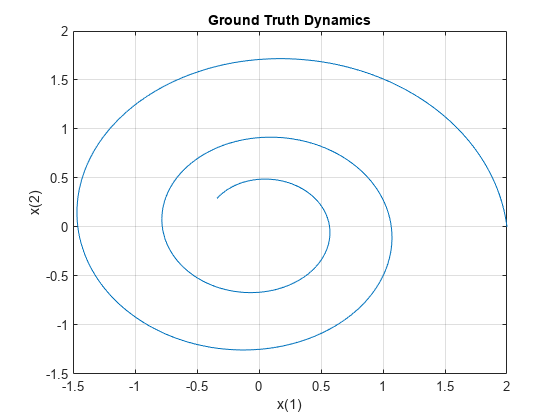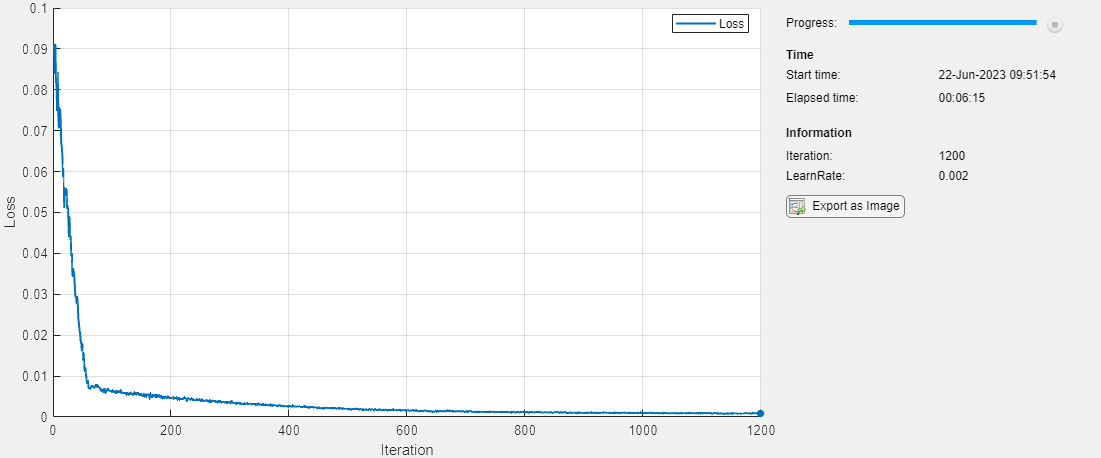# Dynamical System Modeling Using Neural ODE

This example shows how to train a neural network with neural ordinary differential equations (ODEs) to learn the dynamics of a physical system.

Neural ODEs  are deep learning operations defined by the solution of an ODE. More specifically, neural ODE is an operation that can be used in any architecture and, given an input, defines its output as the numerical solution of the ODE

`${y}^{\prime }=f\left(t,y,\theta \right)$`

for the time horizon $\left({t}_{0},{t}_{1}\right)$ and the initial condition $y\left({t}_{0}\right)={y}_{0}$. The right-hand side $f\left(t,y,\theta \right)$ of the ODE depends on a set of trainable parameters $\theta$, which the model learns during the training process. In this example, $f\left(t,y,\theta \right)$ is modeled with a model function containing fully connected operations and nonlinear activations. The initial condition ${y}_{0}$ is either the input of the entire architecture, as in the case of this example, or is the output of a previous operation.

This example shows how to train a neural network with neural ODEs to learn the dynamics $x$ of a given physical system, described by the following ODE:

${x}^{\prime }=Ax$,

where $A$ is a 2-by-2 matrix.

The neural network of this example takes as input an initial condition and computes the ODE solution through the learned neural ODE model.The neural ODE operation, given an initial condition, outputs the solution of an ODE model. In this example, specify a block with a fully connected layer, a tanh layer, and another fully connected layer as the ODE model.In this example, the ODE that defines the model is solved numerically with the explicit Runge-Kutta (4,5) pair of Dormand and Prince . The backward pass uses automatic differentiation to learn the trainable parameters $\theta$ by backpropagating through each operation of the ODE solver.

The learned function $f\left(t,y,\theta \right)$ is used as the right-hand side for computing the solution of the same model for additional initial conditions.

### Synthesize Data of Target Dynamics

Define the target dynamics as a linear ODE model ${x}^{\prime }=Ax$, with `x0` as its initial condition, and compute its numerical solution `xTrain` with `ode45` in the time interval `[0 15]`. To compute an accurate ground truth data, set the relative tolerance of the `ode45` numerical solver to ${10}^{-7}$. Later, you use the value of `xTrain` as ground truth data for learning an approximated dynamics with a neural ODE model.

```x0 = [2; 0]; A = [-0.1 -1; 1 -0.1]; trueModel = @(t,y) A*y; numTimeSteps = 2000; T = 15; odeOptions = odeset(RelTol=1.e-7); t = linspace(0, T, numTimeSteps); [~, xTrain] = ode45(trueModel, t, x0, odeOptions); xTrain = xTrain';```

Visualize the training data in a plot.

```figure plot(xTrain(1,:),xTrain(2,:)) title("Ground Truth Dynamics") xlabel("x(1)") ylabel("x(2)") grid on```### Define and Initialize Model Parameters

The model function consists of a single call to `dlode45` to solve the ODE defined by the approximated dynamics $f\left(t,y,\theta \right)$ for 40 time steps.

```neuralOdeTimesteps = 40; dt = t(2); timesteps = (0:neuralOdeTimesteps)*dt;```

Define the learnable parameters to use in the call to `dlode45` and collect them in the variable `neuralOdeParameters`. The function `initializeGlorot` takes as input the size of the learnable parameters `sz` and the number of outputs and number of inputs of the fully connected operations, and returns a `dlarray` object with underlying type `single` with values set using Glorot initialization. The function `initializeZeros` takes as input the size of the learnable parameters, and returns the parameters as a `dlarray` object with underlying type `single`. The initialization example functions are attached to this example as supporting files. To access these functions, open this example as a live script. For more information about initializing learnable parameters for model functions, see Initialize Learnable Parameters for Model Function.

Initialize the parameters structure.

`neuralOdeParameters = struct;`

Initialize the parameters for the fully connected operations in the ODE model. The first fully connected operation takes as input a vector of size `stateSize` and increases its length to `hiddenSize`. Conversely, the second fully connected operation takes as input a vector of length `hiddenSize` and decreases its length to `stateSize`.

```stateSize = size(xTrain,1); hiddenSize = 20; neuralOdeParameters.fc1 = struct; sz = [hiddenSize stateSize]; neuralOdeParameters.fc1.Weights = initializeGlorot(sz, hiddenSize, stateSize); neuralOdeParameters.fc1.Bias = initializeZeros([hiddenSize 1]); neuralOdeParameters.fc2 = struct; sz = [stateSize hiddenSize]; neuralOdeParameters.fc2.Weights = initializeGlorot(sz, stateSize, hiddenSize); neuralOdeParameters.fc2.Bias = initializeZeros([stateSize 1]);```

Display the learnable parameters of the model.

`neuralOdeParameters.fc1`
```ans = struct with fields: Weights: [20×2 dlarray] Bias: [20×1 dlarray] ```
`neuralOdeParameters.fc2`
```ans = struct with fields: Weights: [2×20 dlarray] Bias: [2×1 dlarray] ```

#### Define Neural ODE Model

Create the function `odeModel`, listed in the ODE Model section of the example, which takes as input the time input (unused), the corresponding solution, and the ODE function parameters. The function applies a fully connected operation, a tanh operation, and another fully connected operation to the input data using the weights and biases given by the parameters.

#### Define Model Function

Create the function `model`, listed in the Model Function section of the example, which computes the outputs of the deep learning model. The function `model` takes as input the model parameters and the input data. The function outputs the solution of the neural ODE.

#### Define Model Loss Function

Create the function `modelLoss`, listed in the Model Loss Function section of the example, which takes as input the model parameters, a mini-batch of input data with corresponding targets, and returns the loss and the gradients of the loss with respect to the learnable parameters.

### Specify Training Options

```gradDecay = 0.9; sqGradDecay = 0.999; learnRate = 0.002;```

Train for 1200 iterations with a mini-batch-size of 200.

```numIter = 1200; miniBatchSize = 200;```

Every 50 iterations, solve the learned dynamics and display them against the ground truth in a phase diagram to show the training path.

`plotFrequency = 50;`

### Train Model Using Custom Training Loop

Initialize the training progress plot.

```f = figure; f.Position(3) = 2*f.Position(3); subplot(1,2,1) C = colororder; lineLossTrain = animatedline(Color=C(2,:)); ylim([0 inf]) xlabel("Iteration") ylabel("Loss") grid on```

Initialize the `averageGrad` and `averageSqGrad` parameters for the Adam solver.

```averageGrad = []; averageSqGrad = [];```

Train the network using a custom training loop.

For each iteration:

• Construct a mini-batch of data from the synthesized data with the `createMiniBatch` function, listed in the Create Mini-Batches Function section of the example.

• Evaluate the model loss and gradients and loss using the `dlfeval` function and the `modelLoss` function, listed in the Model Loss Function section of the example.

• Update the model parameters using the `adamupdate` function.

• Update the training progress plot.

```numTrainingTimesteps = numTimeSteps; trainingTimesteps = 1:numTrainingTimesteps; plottingTimesteps = 2:numTimeSteps; start = tic; for iter = 1:numIter % Create batch [X, targets] = createMiniBatch(numTrainingTimesteps, neuralOdeTimesteps, miniBatchSize, xTrain); % Evaluate network and compute loss and gradients [loss,gradients] = dlfeval(@modelLoss,timesteps,X,neuralOdeParameters,targets); % Update network [neuralOdeParameters,averageGrad,averageSqGrad] = adamupdate(neuralOdeParameters,gradients,averageGrad,averageSqGrad,iter,... learnRate,gradDecay,sqGradDecay); % Plot loss subplot(1,2,1) currentLoss = double(loss); addpoints(lineLossTrain,iter,currentLoss); D = duration(0,0,toc(start),Format="hh:mm:ss"); title("Elapsed: " + string(D)) drawnow % Plot predicted vs. real dynamics if mod(iter,plotFrequency) == 0 || iter == 1 subplot(1,2,2) % Use ode45 to compute the solution y = dlode45(@odeModel,t,dlarray(x0),neuralOdeParameters,DataFormat="CB"); plot(xTrain(1,plottingTimesteps),xTrain(2,plottingTimesteps),"r--") hold on plot(y(1,:),y(2,:),"b-") hold off xlabel("x(1)") ylabel("x(2)") title("Predicted vs. Real Dynamics") legend("Training Ground Truth", "Predicted") drawnow end end```### Evaluate Model

Use the model to compute approximated solutions with different initial conditions.

Define four new initial conditions different from the one used for training the model.

```tPred = t; x0Pred1 = sqrt([2;2]); x0Pred2 = [-1;-1.5]; x0Pred3 = [0;2]; x0Pred4 = [-2;0];```

Numerically solve the ODE true dynamics with `ode45` for the four new initial conditions.

```[~, xTrue1] = ode45(trueModel, tPred, x0Pred1, odeOptions); [~, xTrue2] = ode45(trueModel, tPred, x0Pred2, odeOptions); [~, xTrue3] = ode45(trueModel, tPred, x0Pred3, odeOptions); [~, xTrue4] = ode45(trueModel, tPred, x0Pred4, odeOptions);```

Numerically solve the ODE with the learned neural ODE dynamics.

```xPred1 = dlode45(@odeModel,tPred,dlarray(x0Pred1),neuralOdeParameters,DataFormat="CB"); xPred2 = dlode45(@odeModel,tPred,dlarray(x0Pred2),neuralOdeParameters,DataFormat="CB"); xPred3 = dlode45(@odeModel,tPred,dlarray(x0Pred3),neuralOdeParameters,DataFormat="CB"); xPred4 = dlode45(@odeModel,tPred,dlarray(x0Pred4),neuralOdeParameters,DataFormat="CB");```

### Visualize Predictions

Visualize the predicted solutions for different initial conditions against the ground truth solutions with the function `plotTrueAndPredictedSolutions`, listed in the Plot True and Predicted Solutions Function section of the example.

```figure subplot(2,2,1) plotTrueAndPredictedSolutions(xTrue1, xPred1); subplot(2,2,2) plotTrueAndPredictedSolutions(xTrue2, xPred2); subplot(2,2,3) plotTrueAndPredictedSolutions(xTrue3, xPred3); subplot(2,2,4) plotTrueAndPredictedSolutions(xTrue4, xPred4);```### Helper Functions

#### Model Function

The `model` function, which defines the neural network used to make predictions, is composed of a single neural ODE call. For each observation, this function takes a vector of length `stateSize`, which is used as initial condition for solving numerically the ODE with the function `odeModel`, which represents the learnable right-hand side $f\left(t,y,\theta \right)$ of the ODE to be solved, as right hand side and a vector of time points `tspan` defining the time at which the numerical solution is output. The function uses the vector `tspan` for each observation, regardless of the initial condition, since the learned system is autonomous. That is, the `odeModel` function does not explicitly depend on time.

```function X = model(tspan,X0,neuralOdeParameters) X = dlode45(@odeModel,tspan,X0,neuralOdeParameters,DataFormat="CB"); end```

#### ODE Model

The `odeModel` function is the learnable right-hand side used in the call to `dlode45`. It takes as input a vector of size `stateSize`, enlarges it so that it has length `hiddenSize`, and applies a nonlinearity function `tanh`. Then the function compresses the vector again to have length `stateSize`.

```function y = odeModel(~,y,theta) y = tanh(theta.fc1.Weights*y + theta.fc1.Bias); y = theta.fc2.Weights*y + theta.fc2.Bias; end```

#### Model Loss Function

This function takes as inputs a vector `tspan`, a set of initial conditions `X0`, the learnable parameters `neuralOdeParameters`, and target sequences `targets`. It computes the predictions with the `model` function, and compares them with the given targets sequences. Finally, it computes the loss and the gradient of the loss with respect to the learnable parameters of the neural ODE.

```function [loss,gradients] = modelLoss(tspan,X0,neuralOdeParameters,targets) % Compute predictions. X = model(tspan,X0,neuralOdeParameters); % Compute L1 loss. loss = l1loss(X,targets,NormalizationFactor="all-elements",DataFormat="CBT"); % Compute gradients. gradients = dlgradient(loss,neuralOdeParameters); end```

#### Create Mini-Batches Function

The `createMiniBatch` function creates a batch of observations of the target dynamics. It takes as input the total number of time steps of the ground truth data `numTimesteps`, the number of consecutive time steps to be returned for each observation `numTimesPerObs`, the number of observations `miniBatchSize`, and the ground truth data `X`.

```function [x0, targets] = createMiniBatch(numTimesteps,numTimesPerObs,miniBatchSize,X) % Create batches of trajectories. s = randperm(numTimesteps - numTimesPerObs, miniBatchSize); x0 = dlarray(X(:, s)); targets = zeros([size(X,1) miniBatchSize numTimesPerObs]); for i = 1:miniBatchSize targets(:, i, 1:numTimesPerObs) = X(:, s(i) + 1:(s(i) + numTimesPerObs)); end end```

#### Plot True and Predicted Solutions Function

The `plotTrueAndPredictedSolutions` function takes as input the true solution `xTrue`, the approximated solution `xPred` computed with the learned neural ODE model, and the corresponding initial condition `x0Str`. It computes the error between the true and predicted solutions and plots it in a phase diagram.

```function plotTrueAndPredictedSolutions(xTrue,xPred) xPred = squeeze(xPred)'; err = mean(abs(xTrue(2:end,:) - xPred), "all"); plot(xTrue(:,1),xTrue(:,2),"r--",xPred(:,1),xPred(:,2),"b-",LineWidth=1) title("Absolute Error = " + num2str(err,"%.4f")) xlabel("x(1)") ylabel("x(2)") xlim([-2 3]) ylim([-2 3]) legend("Ground Truth","Predicted") end```

 Chen, Ricky T. Q., Yulia Rubanova, Jesse Bettencourt, and David Duvenaud. “Neural Ordinary Differential Equations.” Preprint, submitted December 13, 2019. https://arxiv.org/abs/1806.07366.

 Shampine, Lawrence F., and Mark W. Reichelt. “The MATLAB ODE Suite.” SIAM Journal on Scientific Computing 18, no. 1 (January 1997): 1–22. https://doi.org/10.1137/S1064827594276424.